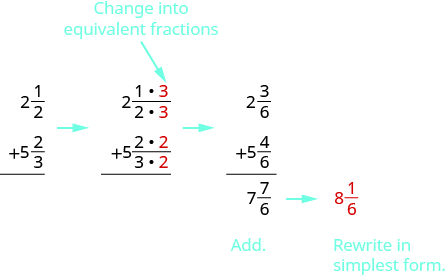## Adding and Subtracting Mixed Numbers With Different Denominators

### Learning Outcomes

• Add and subtract mixed numbers with unlike denominators

To add or subtract mixed numbers with different denominators, we first convert the fractions to equivalent fractions with the LCD. Then we can follow all the steps we have learned for adding or subtracting fractions with like denominators.

In our first video, we show the process of adding mixed numbers with different denominators using pattern blocks.

Now let’s try adding mixed numbers with different denominators without the help of pattern blocks.

### Example

Add: $2\Large\frac{1}{2}+\normalsize5\Large\frac{2}{3}$

Solution:
Since the denominators are different, we rewrite the fractions as equivalent fractions with the LCD, $6$. Then we will add and simplify.We write the answer as a mixed number because we were given mixed numbers in the problem.

### Example

Subtract: $4\Large\frac{3}{4}-\normalsize2\Large\frac{7}{8}$

### Example

Subtract: $3\Large\frac{5}{11}-\normalsize4\Large\frac{3}{4}$

### Try It

In the following video we show four more examples of adding and subtracting mixed numbers that have both like and unlike denominators. The method used for these examples employs improper fractions.